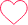# TCS Coding look at the series below: 1,2,3,5,8,13,21,34,55,89,144,233,377,610,987,….. This series is formed as below: 1.term(1)=1

Question 6

look at the series below: 1,2,3,5,8,13,21,34,55,89,144,233,377,610,987,…..

This series is formed as below: 1.term(1)=1

2.term(2)=2

3.term(N)=term(N-1)+term(N-2)for N>2

Write a program to find the Nth term in this series .the value N is a positive integer that should be read from STDIN.the Nth term that is calculated by the program should be written to STDOUT,other than the value of nth term no other characters /strings and messages should be written to STDOUT.

For example if N =15,the value of 15thn term is 987 which is the sum of 13th and 14th terms . You can assume that the value of n will not exceed 30

Ans:

#include <stdio.h>

int main() {

//code int n;

scanf(“%d”, &n);

if(n == 1)

{

printf(“1”);

}

else if(n ==2)

{

printf(“2”);

}

else

{

int t1=1, t2=2, nth_term; for(int i = 3; i <=n; i++)

{

nth_term = t1 + t2; t1 = t2;

t2 = nth_term;

}

printf(“%d”, nth_term);

}

return 0;

}

Please comment the code in other languages as well.

### One comment on “TCS Coding look at the series below: 1,2,3,5,8,13,21,34,55,89,144,233,377,610,987,….. This series is formed as below: 1.term(1)=1”

•61_NAMIRA

Python0